Choose Language Hide Translation Bar
Highlighted

## Odds ratio for a simple distribution

Hi

I'm looking at the distribution of individuals participating in different events. Here, I'm looking at "thirds" (i.e., first, second, third part of the year, not sure "Tertial" is the correct word in english), could also be quarters, Q1-Q4.

When doing a distribution plot, I can also test the probability/ do a chi-2 test, and thereafter get the likelihood ratio, and its confidence intervals. However, is there a easy way of calculating the Odds Ratio for this? That is, the odds that you are placed (in this case, born) in T1 instead of T3. I've tried to look around and one can doo Odds ratios under logistic regression models but that seems inappropiate for this case?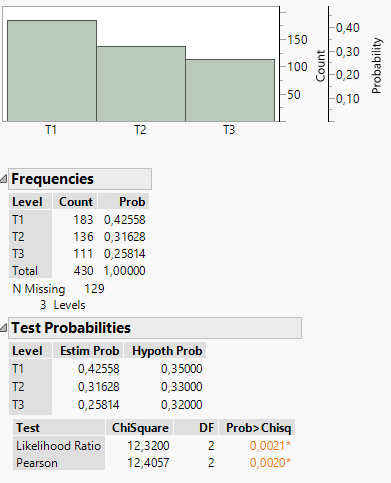15 REPLIES 15
Highlighted

## Re: Odds ratio for a simple distribution

You question is not clear. You have the counts for three groups. You can compute the marginal probability of a group. You can calcualte the odds of a group. The odds ratio would require a covariate or second variable. Do you have another set of three groups for which the external variable is different?

Learn it once, use it forever!
Highlighted

## Re: Odds ratio for a simple distribution

I can imagine!

I have alot of sets with three groups, but mostly I want to calculate the odds that you are in, for example, T1 to T3.

It's quite common practice in the context of Relative Age Effects (in sports, or other fields), in original studies and meta-analysis to calculate the odds ratio, when looking at the [skewed] distribution of participants in sport: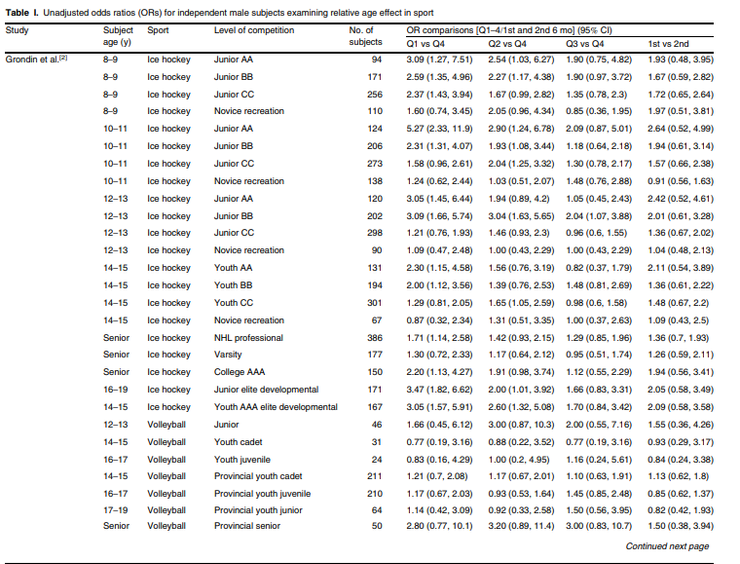I want to to the same.

Highlighted

## Re: Odds ratio for a simple distribution

I will let someone else who knows more than I do. JMP does not compute the odds, as far as I know. You can calculate them from the probability estimates in Distribution that were used to test probabilities.

More could be done with scripting, but you didn't mention that option. I assumed that you were looking for an interactive solution or a solution with a column formula.

On the other hand, if you set up the example (above) in a JMP data table so you have Subject Age (group), Sport, Level of Competition, and the frequency, you can fit any logistic model you want, save the probability formulas, and then compute odds and odds ratios with table summaries and column formulas.

Learn it once, use it forever!
Highlighted

## Re: Odds ratio for a simple distribution

Hm.. So to calculate OR using my probability results (as examplified in first post) would be, for T1 to T3:

( 0.426 / (1-0.426) ) / ( 0.258 / (1- 0.258) ) = 2.13 ?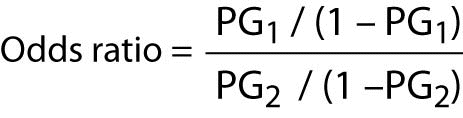Will try your second option, but then I need to re-arrange all the data and type in frequencies etc..

Highlighted

## Re: Odds ratio for a simple distribution

Yes, that is how you compute the odds and odds ratio, although I have never used it this way.

Learn it once, use it forever!
Highlighted

## Re: Odds ratio for a simple distribution

So, would it be possible to calculate Odds ratio (i.e. T1 vs T3) with this dataset? Never used logistic modelling..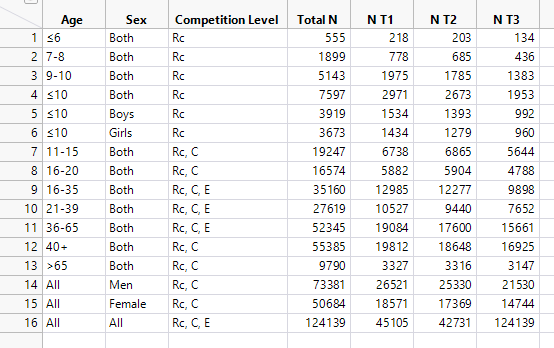Highlighted

## Re: Odds ratio for a simple distribution

You can use the Tables > Stack command to combine the three data columns N T1 through N T3 into one column and create a second label column to capture the original column name. Use the label column for the response and the stacked data column for the frequency. You do not need the total counts for the analysis.

The logistic regression would allow you to model dependencies of the probability on the other variables. The conditional probabilities, in turn, could be used to compute the odds and odds ratios (e.g., different age groups) as you have done above.

Learn it once, use it forever!
Highlighted

## Re: Odds ratio for a simple distribution

Thanks. Almost there...

I stack the data & fits the model. Confused. Is it possible to do the model for the whole dataset? In addition do analysis by age group.

Im not really getting the results table, or finding any odds ratios.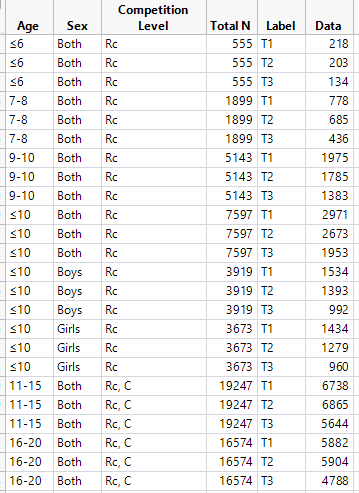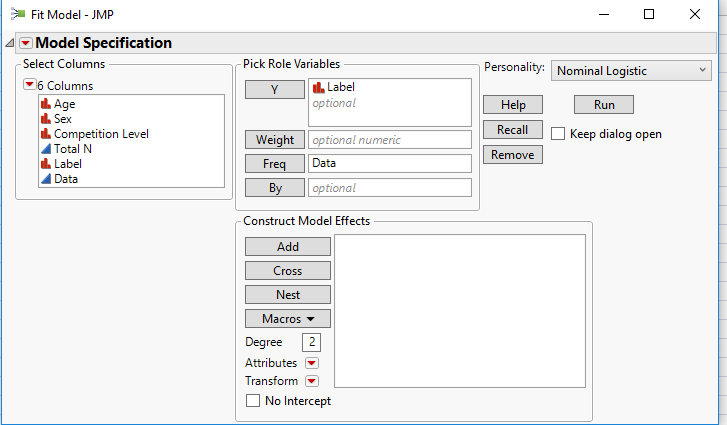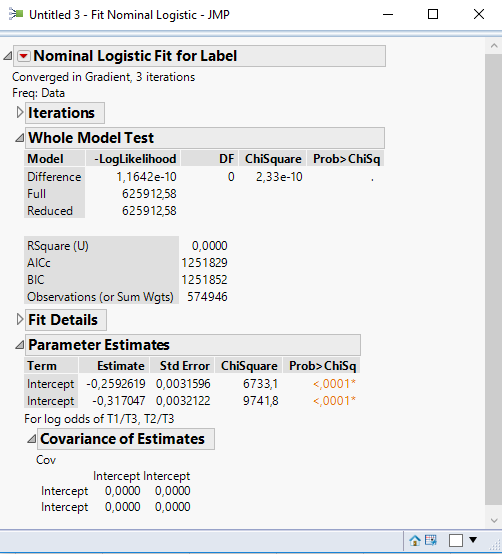Highlighted

## Re: Odds ratio for a simple distribution

You are there but you still have to compute the odds and odds ratios yourself. This method is just another way to get the marginal probabilities (red triangle > Save Probabilities). But it gets more interesting and useful if you want to compute conditional probabilities. Nominal Logistic still does not produce the odds or odds ratios, but you can save the probabilities and then use Tables > Summary to get a concise table of probs for the remaining manual computation.

Learn it once, use it forever!
Article Labels

There are no labels assigned to this post.